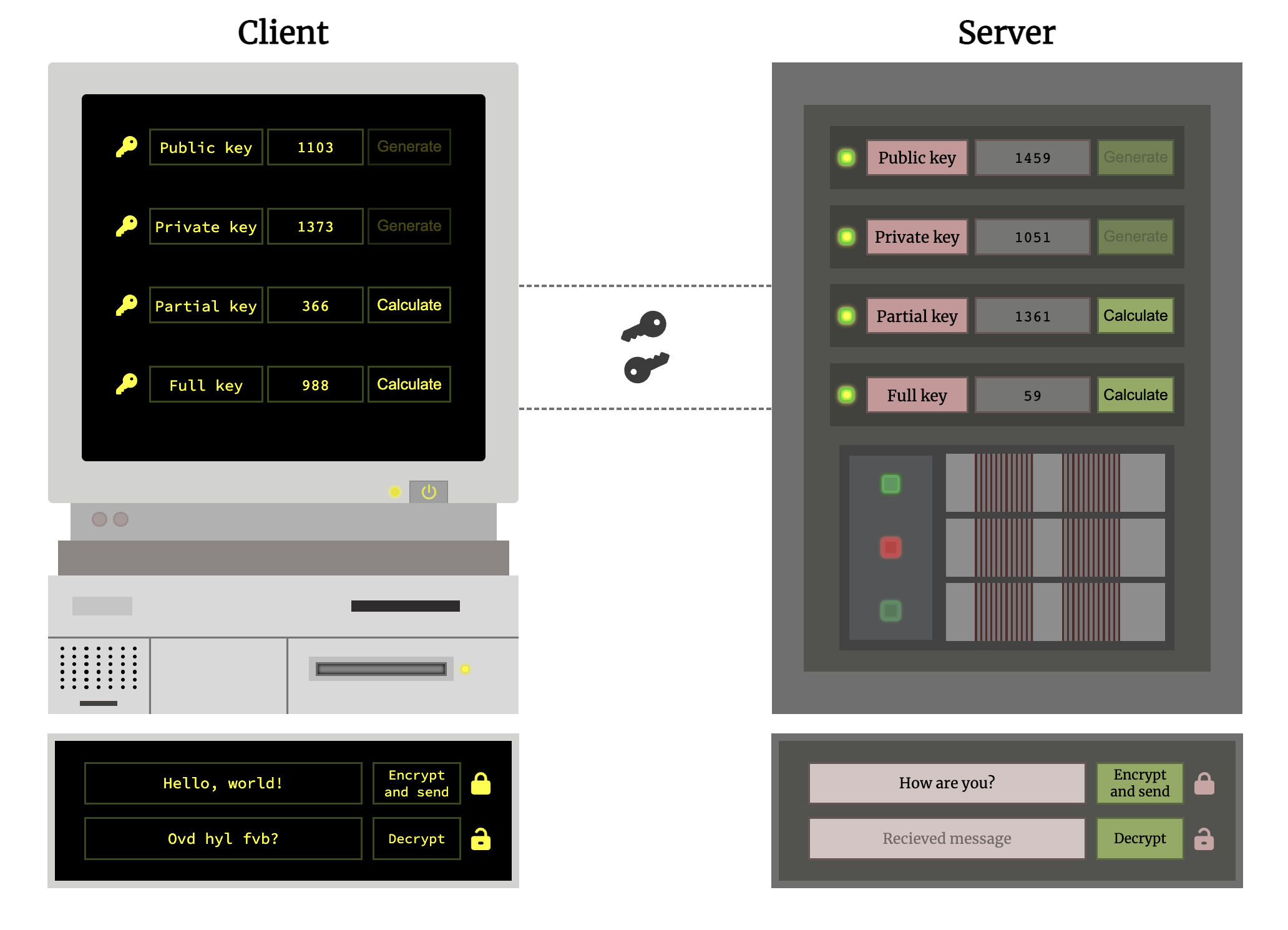# Diffie-Hellman key exchange simulator

This project allows you to manually generate and calculate keys which are used in Diffie-Hellman algorithm. Than you can encrypt, send and decrypt messages. All with fancy animations!

Consider that this is a basic implementation of this algorithm which shall not be used for any real-life encryption purposes!## Introduction

Diffie-Hellman key exchange, also called exponential key exchange, is a method of digital encryption that uses numbers raised to specific powers to produce decryption keys on the basis of components that are never directly transmitted, making the task of a would-be code breaker mathematically overwhelming.

## How does it work?

Let’s imagine that we have two end users – Client and Server. There is a mutual agreement on positive prime numbers between 1000 and 2000 which will be randomly generated and used as public and private keys.

Steps:

• First of all you need to generate public keys for Client and Server. Press `Generate` button next to the `Public key` title and you will see that a random prime number has appeared in the center textarea.
• Next you need to do the same thing but with private keys. Consider that DH KE algorithm implies that public keys are safe to be distributed through unsecured channel and private keys must be kept in secret by end users.
• Now you are ready to calculate partial keys. They are also safe to exchange in unsecured channel because they are still not representing any secure information. Here you can see formulas with which it will be done automatically by pressing `Calculate` button next to the `Partial key` title (c – Client, s – Server):
``````c_partialKey = c_publicKey ** c_privateKey % s_publicKey
``````
``````s_partialKey = c_publicKey ** s_privateKey % s_publicKey
``````
• Last and the most important step – calculate full keys. With them you will encrypt and decrypt messages down below. Here you can see calculating formulas:
``````c_fullKey = s_partialKey ** c_privateKey % s_publicKey
``````
``````s_fullKey = c_partialKey ** s_privateKey % s_publicKey
``````

I guess you’ve noticed that Server full key is totally equal to Client full key. Now both end users have same key which is unknown even to imaginary hacker (because private keys were not distributed here) who has access to all data which was exchanged in this channel.

That was basic explanation but you can take a really deep dive into DH key exchange process here.

## Message encryption and decryption

Now, when all keys are set, you are ready to encrypt and send you first message. Full key will be used for both encryption and decryption. But I guess you have a question – how data will be actually transformed?

For this purpose I’ve also implemented Caesar Shift algorithm here. The Caesar Cipher technique is one of the earliest and simplest method of encryption technique. It’s simply a type of substitution cipher, i.e., each letter of a given text is replaced by a letter some fixed number of positions down the alphabet.Here you can see some examples:

``````abcd (shift = 1) -> bcde
abcd (shift = 2) -> cdef
abcd (shift = 27) -> bcde
``````

You can also describe it with this two formulas (where first is for encryption phase and second is for decryption phase):

``````En(x) = (x + n) % 26
En(x) = (x - n) % 26
``````

As you might have been already noticed `26` here stands for number of letters in English alphabet.

That’s how messages will be encrypted and decrypted. You can type some words in Client or Server messenger box and pres `Encrypt and send`. After a second you will see encrypted message in the opposite messenger box where you can decrypt it by pressing `Decrypt`.

## Conclusion

In this project I’ve implemented a basic version of Diffie-Hellman key exchange algorithm, which can be quite usefull for those who wants to have a real-life example of this process right on their screen.

I’ve also made some pretty complex custom animations to make this proccess funnier and more visual and developed pop-up warning window to make sure that message won’t be decrypted twice.

You can check this app live on GitHub Pages here.

View Github